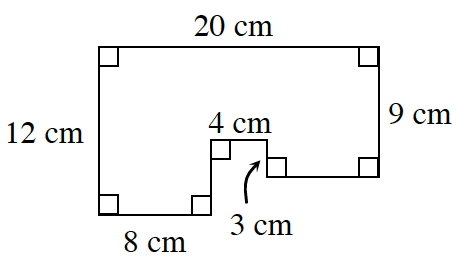### Home > CCG > Chapter 8 > Lesson 8.1.4 > Problem8-46

8-46.At right is a scale drawing of the floor plan for Nzinga’s dollhouse. The actual dimensions of the dollhouse are $5$ times the measurements provided in the floor plan at right.

1. Use the measurements provided in the diagram to find the area and perimeter of her floor plan.

Divide the diagram into three separate rectangles.
Find the area of each individual rectangle, then add them all together.
Afterwards, find the perimeter.

2. Draw a similar figure on your paper. Label the sides with the actual measurements of Nzinga’s dollhouse. What is the perimeter and area of the floor of her actual dollhouse? Show all work.

1.Find the ratio of the perimeters of the two figures. What do you notice?

2. Find the ratio of the areas of the two figures. How does the ratio of the areas seem to be related to the zoom factor?

Find the perimeter of the actual house remember it is $5$ times as big, how does the ratio of the actual house compare to the model house?
Find the areas using the same method in part (a), how does the ratio of the actual house compare to the model house?